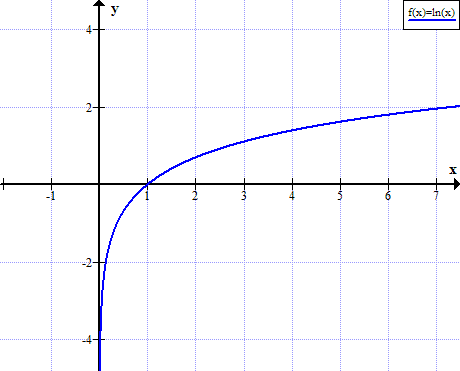# Graph of ln(x)

ln(x) function graph. Natural logarithm graph.

y = f (x) = ln(x)### ln(x) graph properties

• ln(x) is defined for positive values of x.
• ln(x) is not defined for real non positive values of x.
• ln(x)<0 for 0<x<1
• ln(x)=0 for x=1
• ln(x)>0 for x>1

ln(x) rules ►